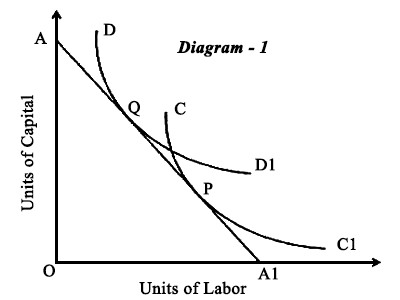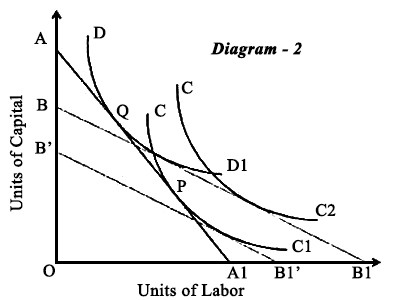# Explain the Heckscher Ohlin Theory of International TradeInternational Trade is the exchange of goods and services between countries. This type of trade gives rise to a world economy, in which prices, supply, and demand are affected by global events. International trade contributes a significant portion for a nations total GOP. Business organizations get a high level of privilege during operating in the international market.

Heckscher Ohlin Theory

The theories of Smith and Ricardo didn’t help countries determine which products would give a country an advantage. Both theories assumed that free and open markets would lead countries and producers to determine which goods they could produce mo efficiently. In the early 1900s, two Swedish economists, Eli Heckscher and Bertil Ohlin, focused their attention on how a country could gain a comparative advantage by producing products that utilized factors that were in abundance in the country. Their theory is based on a country’s production factors – land, labor, and capital, which provide the funds for investment in plants and equipment. They determined that the cost of any factor or resource was a function of supply and demand. Factors that were in great supply relative to demand would be cheaper; factors in great demand relative to supply would be more expensive. Their theory also called the factor proportions theory, stated that countries would produce and export goods that required resources or factors that were in great supply, and, therefore, cheaper production factors. In contrast, countries would import goods that required resources that were in short supply, but higher demand.

For example, China and India are home to cheap, large pools of labor. Hence these countries have become the optimal locations for labor-intensive industries like textiles and garments.

Heckscher Ohlin Theory states that the differences in costs of production between two countries would arise primarily on account of the differences in the factor endowments. The theory can be explained as follows –

Assumptions –

• We assume two countries (Country A and B) and two commodities,
• The relative prices of labor and capital in the two countries will also be different.
• For producing each of the two commodities requires different proportions of labor and capital, i.e., different production functions for different commodities.
• Production function displays constant returns to scale for both commodities.
• Factors of production cannot be moved across countries.
• While there are 2 factors of production, each of the factors is homogenous i.e. 1 unit of labor is similar to the other and so also for capital.
• All factors of production are fully employed and there is perfect competition in both countries.
• No currency.
• No restriction on foreign trade.
• No change in technology. Further technology is the same in both countries.

Explanation – Given the above assumptions the H-O Theory can be explained using the following diagram-Suppose a situation as given in diagram -1

X-axis measures units of Labor while Y-axis measures units of capital.

DD1 is the isoquant curve representing different combinations of capital and labor required to produce units of Bread. CC1 is the isoquant curve for production of units of Cloth. Clearly, as BB 1 is closer to Y- axis indicates that production function for bread is more capital intensive while that of cloth is labor intensive.

Let AA1 be a resource constraint for country A. Given the resources the producer country can employ a maximum of OA units of capital or OA1 units of labor. The slope of the resource constraint line is determined by the relative prices of labor and capital.

With the given isoquants and the resource constraint, the country is in a position to produce Bread at point Q and Cloth at point P as these are the points at which both die isoquants are tangential to the resource constraint.

Now assume a situation as in diagram -2

where there is a change in the relative prices of labor and capital. Say a country B which has abundant labor. The slope of the resource constraint changes to BB1.It can be seen that with the resource constraint slope at BB1, the country can produce units of Bread on DD1. However, it can produce a higher amount of cloth at CC2 with the same amount of resources.

On the other hand, it can produce the original quantity of cloth CC1 with much lesser resources BB1.

It is clear thus that as country B is abundant in labor, it is more efficient in the production of cloth, which is a labor-intensive commodity. Country B would thus specialize in the production of cloth and export cloth to country A.

Whereas, country A, being a capital abundant country, would be more efficient in the production of Bread and end up exporting bread to country B.